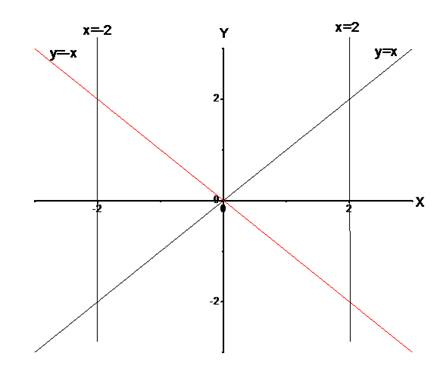# The graph for the given equation.### Single Variable Calculus: Concepts...

4th Edition
James Stewart
Publisher: Cengage Learning
ISBN: 9781337687805### Single Variable Calculus: Concepts...

4th Edition
James Stewart
Publisher: Cengage Learning
ISBN: 9781337687805

#### Solutions

Chapter 1, Problem 8P
To determine

## To find: The graph for the given equation.

Expert Solution

### Explanation of Solution

Given:

The given equation is x44x2x2y2+4y2=0 .

Calculation:

Consider the given equation is,

x44x2x2y2+4y2=0

Then,

x44x2x2y2+4y2=0x2(x24)(x2y2)=0(x2)(x+2)(xy)(x+y)=0

Then, the zeroes of the above equation are,

y=xy=xx=2x=2

The graph for the equation is shown in Figure 1Figure 1

### Have a homework question?

Subscribe to bartleby learn! Ask subject matter experts 30 homework questions each month. Plus, you’ll have access to millions of step-by-step textbook answers!